# For the hook support shown, determine by trigonometry the magnitude and direction of the resultant of the two forces applied to the hook

Question-AnswerCategory: Engineering MechanicsFor the hook support shown, determine by trigonometry the magnitude and direction of the resultant of the two forces applied to the hook

For the hook support shown, determine by trigonometry the magnitude and direction of the resultant of the two forces applied to the hook.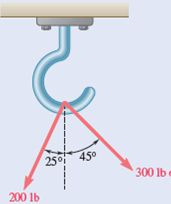Step No: 1

Draw a triangle with the forces as shown: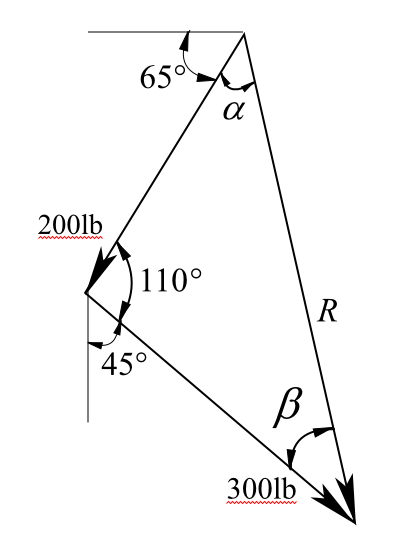Here, R is the resultant of the two forces.

Step No: 2

Apply law of cosines to find the resultant R.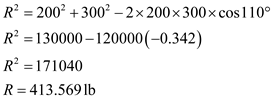Therefore, the resultant of two forces applied to the hook is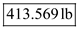.

Step No: 3

Apply sine rule to find the direction of resultant force.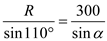Substitute 413.569lb for R.Find the angle of the resultant with respect to the horizontal.Substitutefor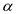.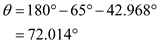Therefore, the angle made by the resultant with the resultant is